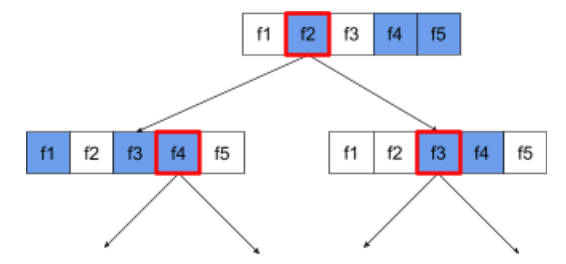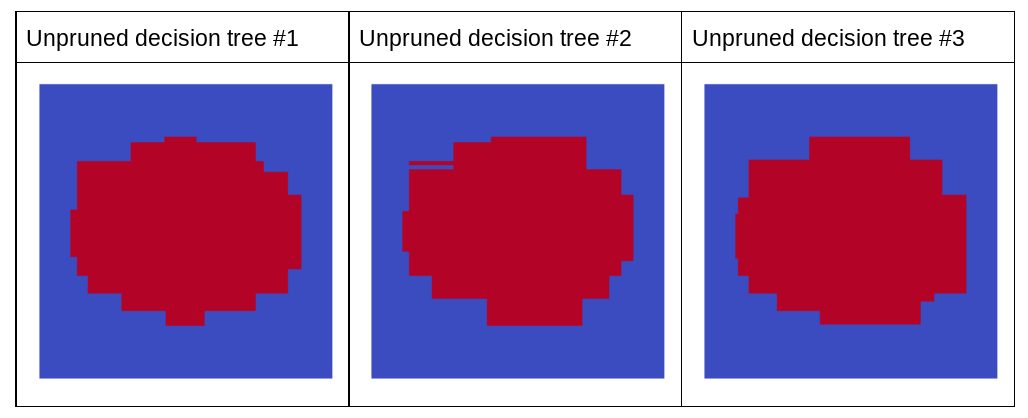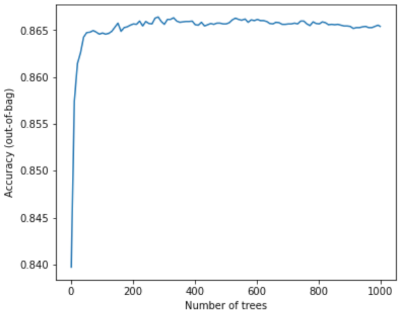## Random forests

A random forest (RF) is an ensemble of decision trees in which each decision tree is trained with a specific random noise. Random forests are the most popular form of decision tree ensemble. This unit discusses several techniques for creating independent decision trees to improve the odds of building an effective random forest.

### Bagging

Bagging (bootstrap aggregating) means training each decision tree on a random subset of the examples in the training set. In other words, each decision tree in the random forest is trained on a different subset of examples.

Bagging is peculiar. Each decision tree is trained on the same number of examples as in the original training set. For example, if the original training set contains 60 examples, then each decision tree is trained on 60 examples. However, bagging only trains each decision tree on a subset (typically, 67%) of those examples. So, some of those 40 examples in the subset must be reused while training a given decision tree. This reuse is called training "with replacement."

For example, Table 6 shows how bagging could distribute six examples across three decision trees. Notice the following:

• Each decision tree trains on a total of six examples.
• Each decision tree trains on a different set of examples.
• Each decision tree reuses certain examples. For example, example #4 is used twice in training decision tree 1; therefore, the learned weight of example #4 is effectively doubled in decision tree 1.

Table 6. Bagging six training examples across three decision trees. Each number represents the number of times a given training example (#1-6) is repeated in the training dataset of a given decision tree (1-3).

training examples
#1 #2 #3 #4 #5 #6
original dataset 1 1 1 1 1 1
decision tree 1 1 1 0 2 1 1
decision tree 2 3 0 1 0 2 0
decision tree 3 0 1 3 1 0 1

In bagging, each decision tree is almost always trained on the total number of examples in the original training set. Training each decision tree on more examples or fewer examples tends to degrade the quality of the random forest.

While not present in the original random forest paper, the sampling of examples is sometimes done "without replacement"; that is, a training example cannot be present more than once in a decision tree training set. For example, in the preceding table, all values would all be either 0 or 1.

You can enable training without replacement with the following assignment in TF-DF: bootstrap_training_dataset=False

### Attribute sampling

Attribute sampling means that instead of looking for the best condition over all available features, only a random subset of features are tested at each node. The set of tested features is sampled randomly at each node of the decision tree.

The following decision tree illustrates the attribute / feature sampling. Here a decision tree is trained on 5 features (f1-f5). The blue nodes represent the tested features while the white ones are not tested. The condition is built from the best tested features (represented with a red outline).Figure 21. Attribute sampling.

The ratio of attribute sampling is an important regularization hyperparameter. The preceding Figure used a ~⅗ ratio. Many random forest implementations test, by default, 1/3 of the features for regression and sqrt(number of features) for classification.

In TF-DF, the following hyperparameters control attribute sampling:

• num_candidate_attributes
• num_candidate_attributes_ratio

For example, if num_candidate_attributes_ratio=0.5, half of the features will be tested at each node.

### Disabling decision tree regularization

The decision trees in a random forest are trained without pruning (as described in Overfitting and pruning). The lack of pruning significantly increases the variance and significantly reduces the bias of the individual decision tree learning. In other words, the individual decision trees overfit, but the random forest is not.

We expect the training and test accuracy of a random forest to differ. The training accuracy of a random forest is generally much higher (sometimes equal to 100%). However, a very high training accuracy in a random forest is normal and does not indicate that the random forest is overfitted.

The two sources of randomness (bagging and attribute sampling) ensure the relative independence between the decision trees. This independence corrects the overfitting of the individual decision trees. Consequently, the ensemble is not overfitted. We'll illustrate this non-intuitive effect in the next unit.

Pure random forests train without maximum depth or minimum number of observations per leaf. In practice, limiting the maximum depth and minimum number of observations per leaf is beneficial. By default, many random forests use the following defaults:

• maximum depth of ~16
• minimum number of observations per leaf of ~5.

You can tune these hyperparameters.

TF-DF provides the following hyperparameters for tuning:
• max_depth: to adjust the maximum depth
• min_examples: to adjust the minimum number of observations

### The clarity of noise

Why would random noise improve the quality of a random forest? To illustrate the benefits of random noise, Figure 22 shows the predictions of a classical (pruned) decision tree and a random forest trained on a few examples of simple two-dimensional problem with an ellipse pattern.

Ellipses patterns are notoriously hard for decision tree and decision forest algorithms to learn with axis-aligned conditions, so they make a good example. Notice that the pruned decision tree can't get the same quality of prediction as the random forest.Figure 22. Ground truth vs. predictions generated by a single pruned decision tree and predictions generated by a random forest.

The next plot shows the predictions of the first three unpruned decision trees of the random forest; that is, the decision trees are all trained with a combination of:

• bagging
• attribute sampling
• disabling pruning

Notice that the individual predictions of these three decision trees are worse than the predictions of the pruned decision tree in the preceding figure. However, since the errors of the individual decision trees are only weakly correlated, the three decision trees combine in an ensemble to create effective predictions.Figure 23. Three unpruned decision trees that will build an effective ensemble.

Because the decision trees of a random forest are not pruned, training a random forest does not require a validation dataset. In practice, and especially on small datasets, models should be trained on all the available data.

When training a random forest, as more decision trees are added, the error almost always decreases; that is, the quality of the model almost always improves. Yes, adding more decision trees almost always reduces the error of the random forest. In other words, adding more decision trees cannot cause the random forest to overfit. At some point, the model just stops improving. Leo Breiman famously said, "Random Forests do not overfit".

For example, the following plot shows the test evaluation of a random forest model as more decision trees are added. The accuracy rapidly improves until it plateaus around 0.865. However, adding more decision trees does not make accuracy decrease; in other words,the model does not overfit. This behavior is (mostly) always true and independent of the hyperparameters.Figure 24. Accuracy stays constant as more decision trees are added to the random forest.

[{ "type": "thumb-down", "id": "missingTheInformationINeed", "label":"Missing the information I need" },{ "type": "thumb-down", "id": "tooComplicatedTooManySteps", "label":"Too complicated / too many steps" },{ "type": "thumb-down", "id": "outOfDate", "label":"Out of date" },{ "type": "thumb-down", "id": "samplesCodeIssue", "label":"Samples / code issue" },{ "type": "thumb-down", "id": "otherDown", "label":"Other" }]
[{ "type": "thumb-up", "id": "easyToUnderstand", "label":"Easy to understand" },{ "type": "thumb-up", "id": "solvedMyProblem", "label":"Solved my problem" },{ "type": "thumb-up", "id": "otherUp", "label":"Other" }]